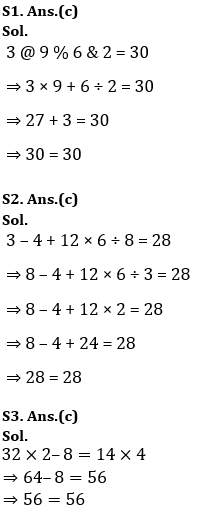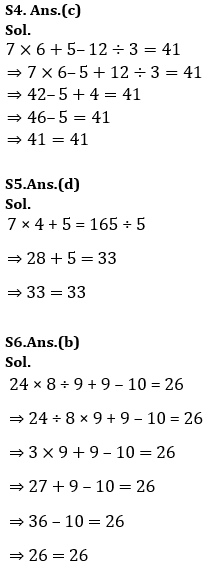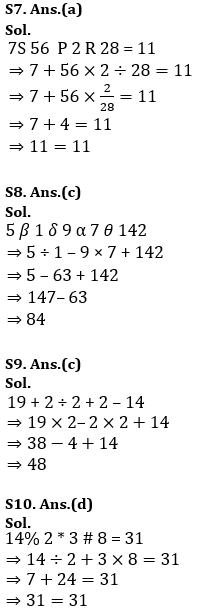# Target SSC CGL | 10,000+ Questions | Reasoning Questions For SSC CGL : Day 122

This is the new year with new goals, new experiences and lots of exams to be scheduled soon. SSC CGL has recently released the exam dates so now it is time to gear up your preparations and try hard to get success. ADDA247 never fails to deliver something new and fruitful for you all. This time also we are providing you the best study plan as well as a study material. We are here going to prepare your Reasoning section for the SSC CGL. In this article, we are providing you the details that how we are going to help you to clear the examination this year. We ADDA247 is going to provide you daily tests for all the subjects. The topic-wise quiz will be done from January till April. This will help you to get a deeper knowledge of all the topics and will prepare you thoroughly.

Q1.If “@” denotes “multiplied by”, “\$” denotes “subtracted from”, “%” denotes “added to” and “&” denotes “divided by”, then which of the following equation must be true?
(a) 7 % 9 & 3 \$ 5 = 4
(b) 4 % 3 @ 6 \$ 9 = 12
(c) 3 @ 9 % 6 & 2 = 30
(d) 6 % 9 @ 2 \$ 7 = 16

Q2.In the following question, correct the equation by interchanging two numbers.
3 – 4 + 12 × 6 ÷ 8 = 28
(a) 3 and 4
(b) 4 and 8
(c) 3 and 8
(d) 4 and 6

Q3.In the following question, by using which mathematical operators will the expression become correct?
32 ? 2 ? 8 ? 14 ? 4
(a) x, +, = and ÷
(b) +, +, = and x
(c) x, –, = and x
(d) +, x, = and x

Q4.In the following question, correct the equation by interchanging two signs.
7 × 6 + 5 – 12 ÷ 3 = 41
(a) + and ÷
(b) × and ÷
(c) + and –
(d) × and –

Q5.In the following question, by using which mathematical operators will the expression become correct?
7 ? 4 ? 5 ? 165 ? 5
(a) +, +, ÷ and =
(b) ×, ×, = and +
(c) ×, ÷, = and ÷
(d) ×, +, = and ÷

Q6.In the following question, correct the equation by interchanging two signs.
24 × 8 ÷ 9 + 9 – 10 = 26
(a) ÷ and –
(b) ÷ and ×
(c) × and –
(d) × and +

Q7.If “P” denotes “multiplied by”, “Q” denotes “subtracted from”, “S” denotes “added to” and “R” denotes “divided by”, then which of the following equation must be true?
(a) 7 S 56 P 2 R 28 = 11
(b) 36 R 6 P 2 S 4 = 19
(c) 64 R 8 P 3 S 6 = 72
(d) 36 R 9 S 4 P 2 = 14

Q8.If “θ” denotes “added to”, “δ” denotes “subtracted from”, “β” denotes “divided by” and “α” denotes “multiplied by”, then 5 β 1 δ 9 α 7 θ 142 = ?
(a) 114
(b) 98
(c) 84
(d) 125

Q9.If “÷” denotes “subtracted from”, “+” denotes “multiplied by”, “–” denotes “added to” and “×” denotes “divided by”, then19 + 2 ÷ 2 + 2 – 14 = ?
(a) 52
(b) 46
(c) 48
(d) 88

Q10.If “#” denotes “multiplied by”, “@” denotes “subtracted from”, “*” denotes “added to” and “%” denotes “divided by”, then which of the following equation must be true?
(a) 4 % 2 # 6 * 7 = 18
(b) 3 # 9 @ 4 # 6 = 4
(c) 5 # 6 % 3 @ 4 = 8
(d) 14 % 2 * 3 # 8 = 31

SolutionsSharing is caring!

June Current Affairs PDF

×

Thank You, Your details have been submitted we will get back to you.Join India's largest learning destination

What You Will get ?

•Job Alerts
•Daily Quizzes
•Subject-Wise Quizzes
•Current Affairs
•Previous year question papers
•Doubt Solving session

ORJoin India's largest learning destination

What You Will get ?

•Job Alerts
•Daily Quizzes
•Subject-Wise Quizzes
•Current Affairs
•Previous year question papers
•Doubt Solving session

ORJoin India's largest learning destination

What You Will get ?

•Job Alerts
•Daily Quizzes
•Subject-Wise Quizzes
•Current Affairs
•Previous year question papers
•Doubt Solving session

Enter the email address associated with your account, and we'll email you an OTP to verify it's you.Join India's largest learning destination

What You Will get ?

•Job Alerts
•Daily Quizzes
•Subject-Wise Quizzes
•Current Affairs
•Previous year question papers
•Doubt Solving session

Enter OTP

Please enter the OTP sent to
/6

Did not recive OTP?

Resend in 60sJoin India's largest learning destination

What You Will get ?

•Job Alerts
•Daily Quizzes
•Subject-Wise Quizzes
•Current Affairs
•Previous year question papers
•Doubt Solving sessionJoin India's largest learning destination

What You Will get ?

•Job Alerts
•Daily Quizzes
•Subject-Wise Quizzes
•Current Affairs
•Previous year question papers
•Doubt Solving session

Almost there

+91Join India's largest learning destination

What You Will get ?

•Job Alerts
•Daily Quizzes
•Subject-Wise Quizzes
•Current Affairs
•Previous year question papers
•Doubt Solving session

Enter OTP

Please enter the OTP sent to Edit Number

Did not recive OTP?

Resend 60

By skipping this step you will not recieve any free content avalaible on adda247, also you will miss onto notification and job alerts

Are you sure you want to skip this step?

By skipping this step you will not recieve any free content avalaible on adda247, also you will miss onto notification and job alerts

Are you sure you want to skip this step?